# [MOD] RNG - Random Number Generator for modders

#### emanueru_95

##### Recruit
•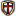•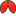•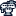•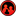•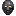•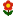•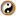•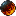•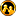•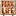•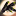•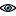•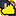•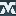•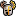•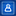•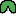•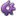•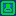•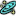•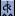•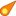•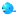•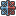•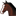•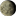•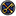•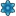•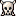•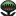•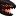•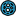•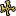•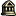•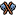•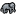•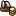•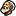•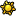•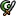•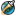I started modding this game for the first time a couple of days ago and needed a random percentage but I didn't find a way to generate random numbers other than creating long lists.
So I wrote my own RNG to create random percents and added a random number generator as a plus if I needed it later. Looks like is working pretty well.

Don't know if the devs will add this feature in the near future but for now this should do the trick.
Feel free to use it in any mod.
Code:
``````# RANDOM NUMBER GENERATOR

# Written by emanueru_95

#############################################################
# Generates a random number between 0.000 and 1.000            #
# Generated percent is saved in variable "rng_percent".        #
#############################################################

rng_percent_effect = {
# Clean variable rng_percent.
set_variable = {
which = rng_percent
value = 0
}
random_list = {
# Chance of rng_percent being EQUAL to 100.0%
1 = {
set_variable = {
which = rng_percent
value = 1
}
}
# Chance of rng_percent being LESS than 100.0%.
1000 = {
while = {
count = 3
limit = {                    #
check_variable = {        #
which = rng_percent    #    Dummy limit
value = 0            #    it doesn't do anything.
}                        #
}
random_list = {
1 = {
change_variable = {
which = rng_percent
value = 0
}
}
1 = {
change_variable = {
which = rng_percent
value = 1
}
}
1 = {
change_variable = {
which = rng_percent
value = 2
}
}
1 = {
change_variable = {
which = rng_percent
value = 3
}
}
1 = {
change_variable = {
which = rng_percent
value = 4
}
}
1 = {
change_variable = {
which = rng_percent
value = 5
}
}
1 = {
change_variable = {
which = rng_percent
value = 6
}
}
1 = {
change_variable = {
which = rng_percent
value = 7
}
}
1 = {
change_variable = {
which = rng_percent
value = 8
}
}
1 = {
change_variable = {
which = rng_percent
value = 9
}
}
}
divide_variable = {
which = rng_percent
value = 10
}
}
}
}
}

#####################################################################################################################
# Generates a random number between "rng_min" and "rng_max" with a specified number of decimals in "rng_places".    #
# Generated number is saved in variable "rng_number".                                                                #
# Variables "rng_max", "rng_min", "rng_places" and "rng_percent" are set to 0 at the end of this effect.            #
# Needs at least "rng_max" OR "rng_min" variable to work properly.                                                    #
#####################################################################################################################

rng_number_effect = {
# Generate random number between "rng_min" and "rng_max".
subtract_variable = {
which = rng_max
which = rng_min
}
rng_percent_effect = yes
multiply_variable = {
which = rng_percent
which = rng_max
}
set_variable = {
which = rng_number
which = rng_percent
}
while = {
count = 2
limit = {
check_variable = {
which = rng_number
value = 0.001
}
}
divide_variable = {
which = rng_max
value = 1000
}
rng_percent_effect = yes
multiply_variable = {
which = rng_percent
which = rng_max
}
subtract_variable = {
which = rng_number
which = rng_percent
}
}
change_variable = {
which = rng_number
which = rng_min
}
# Round generated number to specified number of places.
if = {
limit = {
check_variable = {
which = rng_places
value == 0
}
}
if = {
limit = {
check_variable = {
which = rng_number
value = 0
}
}
change_variable = {
which = rng_number
value = 0.5
}
}
else = {
change_variable = {
which = rng_number
value = -0.5
}
}
divide_variable = {
which = rng_number
value = 1000
}
multiply_variable = {
which = rng_number
value = 1000
}
}
else_if = {
limit = {
check_variable = {
which = rng_places
value == 1
}
}
if = {
limit = {
check_variable = {
which = rng_number
value = 0
}
}
change_variable = {
which = rng_number
value = 0.05
}
}
else = {
change_variable = {
which = rng_number
value = -0.05
}
}
divide_variable = {
which = rng_number
value = 100
}
multiply_variable = {
which = rng_number
value = 100
}
}
else_if = {
limit = {
check_variable = {
which = rng_places
value == 2
}
}
if = {
limit = {
check_variable = {
which = rng_number
value = 0
}
}
change_variable = {
which = rng_number
value = 0.005
}
}
else = {
change_variable = {
which = rng_number
value = -0.005
}
}
divide_variable = {
which = rng_number
value = 10
}
multiply_variable = {
which = rng_number
value = 10
}
}
# Clean variables.
set_variable = {
which = rng_max
value = 0
}
set_variable = {
which = rng_min
value = 0
}
set_variable = {
which = rng_places
value = 0
}
set_variable = {
which = rng_percent
value = 0
}
}``````

HOW TO USE:
Code:
``````# Random percentage generator (0.000 to 1.000) ----------------------------------

rng_percent_effect = yes

set_variable = {
which = <myvar>
which = rng_percent
}

# Random number generator (rng_min to rng_max)-------------------------------------------
# Maximun number to be generated, 0 if not specified
set_variable = {
which = rng_max
which = <myvar1>
}
# Minimun number to be generated, 0 if not specified
set_variable = {
which = rng_min
which = <myvar2>
}
# Number of decimals for the generated number from 0 to 3, 0 if not specified (Returns integers)
set_variable = {
which = rng_places
value = 2
}

rng_number_effect = yes

set_variable = {
which = <myvar3>
which = rng_number
}``````

#### Attachments

• RNG.zip
1,3 KB · Views: 5

#### corsairmarks

##### Corporal
•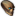•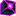•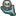••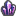••••••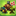••••••••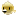•Thank you for this nice piece of code! I modified it for use with Stellaris.